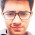Probabilities and drawing ping pong balls - FreeEconHelp.com, Learning Economics... Solved!

## 3/9/12

This post will go over probability theory.   While not directly related to economics, probabilities and statistics are used in economics quite a bit.  Here is the question giving people problems:

There are two bags, each containing 1000 ping-pong balls. Bag A contains 1000 red balls and no black balls, and bag B contains 200 red and 800 black. You are blindfolded and reach into a bag. There is a .5 probability it is bag A and a .5 probability it is bag B. You draw a red ball. What is the probability that you drew the ball from bag A.

`The math for doing probabilities goes like this.  If you use the word AND you use X in the calculation. `
`If you use the word OR then you use + in the calculation.`

For this particular problem you just need to multiply the probabilities by each other.  Note the sentence:

You drew from bag A AND (X) a red ball.

So the probability of getting the first bag is 1/2, and the probability of getting a red ball in the bag is 1000/1000 or 1, so multiplying them together gives you 1/2 or 5/10.  Let's go through the other scenarios:

You drew from bag A AND a black ball.

The probability of the first bag is 1/2, and the probability of getting a black ball from that bag is 0, so multiplying them together gives 0.

You drew from bag B AND a red ball.

The probability of the second bag is 1/2, and the probability of getting a red ball from that bag is 200/1000 or 1/5, and multiplying those together gives 1/10.

You drew from bag B AND a black ball.

The probability of the second bag is 1/2, and the probability of getting a black ball from that bag is 800/1000 or 4/5, and multiplying those together gives 4/10.

When we add all of the possibilities together we should get 1 (because we KNOW there will be an outcome) and we get 5/10 plus 0 plus 1/10 plus 4/10 added together gives us 1.  So we know we did everything right.

If we wanted to know the probability of drawing from bag A or drawing a black ball we would have to add the probabilities together.  The probability of drawing from bag A is 1/2, and the probability of drawing a black ball is 4/10 (from adding 0 to 4/10 from above).

So the final probability of this state will be 5/10 plus 4/10 or 9/10.

1.Children love playing with balls. Ping pong balls are great for a variety of fun activities. Here a few you can try out with your baby today. table tennis rules and regulations

2.Additionally there are novices who begin with bunches of expectation, just to find later they can't keep up their concentration or essentially attracting does not appear to be that captivating to them as it had appeared before beginning, as a result of the immense size of the expectation to absorb information. Paul Marciano

3.You have shared a very informative article here about the Probability. After reading your article I got very much information and it resolved many of my doubts. If anyone looking for the Online Statistics Tutors, Visit TutorDip

4.types. Pool and snooker cues common round fifty seven–fifty nine inches (140–a hundred and fifty cm) in length and are of three fundamental kinds. look here

5.most of the forty+ pool cue manufacturers we stock are: action, Cuetec, Lucasi, McDermott, Meucci and Viking. table tennis tricks to win

6.ing Pong Table Reviews. When you're prepared to bring the fun and fervor of ping pong into the solace of you more helpful hints

7.This is a great blog that explains the probabilities of drawing of Ping pong balls. If you are looking to buy stacking conference chairs you can buy them at great price online by clicking on this link.